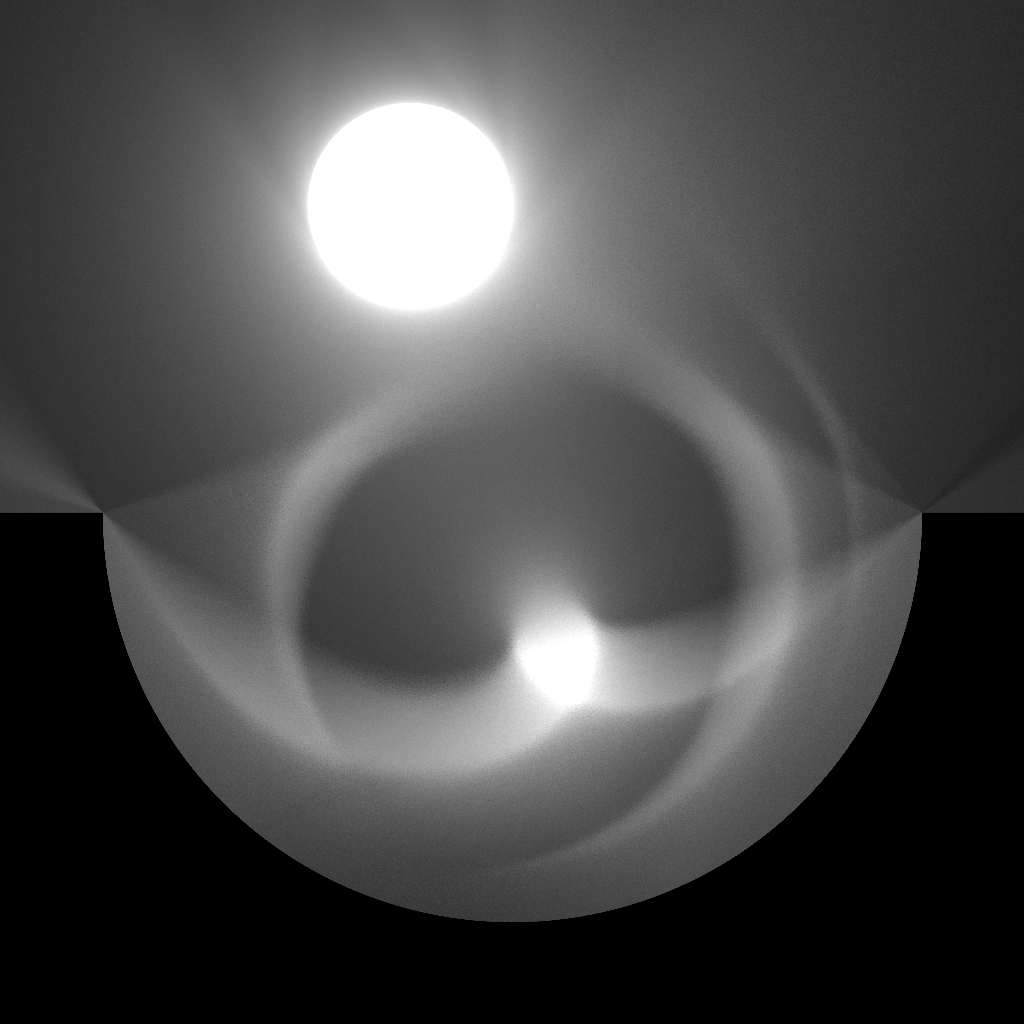## 1. 反射矢量void reflect(float ix, float iy, float nx, float ny, float* rx, float* ry) {
float idotn2 = (ix * nx + iy * ny) * 2.0f;
*rx = ix - idotn2 * nx;
*ry = iy - idotn2 * ny;
}

## 2. 梯度与法线void gradient(float x, float y, float* nx, float* ny) {
*nx = (scene(x + EPSILON, y).sd - scene(x - EPSILON, y).sd) * (0.5f / EPSILON);
*ny = (scene(x, y + EPSILON).sd - scene(x, y - EPSILON).sd) * (0.5f / EPSILON);
}

Result scene(float x, float y) {
Result a = {  circleSDF(x, y, 0.4f,  0.2f, 0.1f), 2.0f };
Result b = {     boxSDF(x, y, 0.5f,  0.8f, TWO_PI / 16.0f, 0.1f, 0.1f), 0.0f };
Result c = {     boxSDF(x, y, 0.8f,  0.5f, TWO_PI / 16.0f, 0.1f, 0.1f), 0.0f };
return unionOp(unionOp(a, b), c);
}

for (int y = 0; y < H; y++)
for (int x = 0; x < W; x++, p += 3) {
float nx, ny;
gradient((float)x / W, (float)y / H, &nx, &ny);
p = (int)((fmaxf(fminf(nx, 1.0f), -1.0f) * 0.5f + 0.5f) * 255.0f);
p = (int)((fmaxf(fminf(ny, 1.0f), -1.0f) * 0.5f + 0.5f) * 255.0f);
p = 0;
}

（注：由于有数值误差，计算出来的梯度分量可能略超出的范围，所以在输出时限制其范围。但这个轻微的误差不影响我们计算反射。）

## 3. 递归追踪

typedef struct { float sd, emissive, reflectivity; } Result;

Result scene(float x, float y) {
Result a = {  circleSDF(x, y, 0.4f,  0.2f, 0.1f), 2.0f, 0.0f };
Result b = {     boxSDF(x, y, 0.5f,  0.8f, TWO_PI / 16.0f, 0.1f, 0.1f), 0.0f, 0.9f };
Result c = {     boxSDF(x, y, 0.8f,  0.5f, TWO_PI / 16.0f, 0.1f, 0.1f), 0.0f, 0.9f };
return unionOp(unionOp(a, b), c);
}

#define BIAS 1e-4f
#define MAX_DEPTH 3

float trace(float ox, float oy, float dx, float dy, int depth) {
float t = 0.0f;
for (int i = 0; i < MAX_STEP && t < MAX_DISTANCE; i++) {
float x = ox + dx * t, y = oy + dy * t;
Result r = scene(x, y);
if (r.sd < EPSILON) {
float sum = r.emissive;
if (depth < MAX_DEPTH && r.reflectivity > 0.0f) {
float nx, ny, rx, ry;
reflect(dx, dy, nx, ny, &rx, &ry);
sum += r.reflectivity * trace(x + nx * BIAS, y + ny * BIAS, rx, ry, depth + 1);
}
return sum;
}
t += r.sd;
}
return 0.0f;
}

float sample(float x, float y) {
float sum = 0.0f;
for (int i = 0; i < N; i++) {
float a = TWO_PI * (i + (float)rand() / RAND_MAX) / N;
sum += trace(x, y, cosf(a), sinf(a), 0); // 开始时深度为 0
}
return sum / N;
}

Result scene(float x, float y) {
Result a = { circleSDF(x, y, 0.4f, 0.2f, 0.1f), 2.0f, 0.0f };
Result d = {  planeSDF(x, y, 0.0f, 0.5f, 0.0f, -1.0f), 0.0f, 0.9f };
Result e = { circleSDF(x, y, 0.5f, 0.5f, 0.4f), 0.0f, 0.9f };
return unionOp(a, subtractOp(d, e));
}